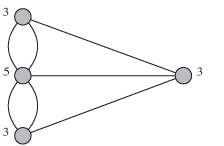Highways - Math Matters - Maplesoft

# Highways

Good highway systems require more than building sturdy roads. To achieve efficient movement of people and goods, scientists and engineers deploy a wide range of mathematical techniques.

Operations Research (OR) was developed by the military. Its goal was to develop a mathematical framework for solving complex logistics problems. Through techniques called mathematical programming, optimization, and others, engineers can design highway networks that balance transportation efficiency with resource constraints.One of the first traffic problems:
Leonhard Euler (Swiss, 1707-1783) introduced a famous math problem called the Seven Bridges of Königsberg – how do you cross over all seven bridges without crossing over any bridge more than once?

To analyze this problem, Euler introduced graph theory, which represents connected elements (like places connected by bridges), as lines and points (edges and vertices) and provides mathematical tools to solve key problems. Through graph theory Euler proved that it is impossible to not cross any bridge more than once. Today, graph theory is an important mathematical technique for designing networks of all kinds including networks of highways.